1. /
2. CBSE
3. /
4. Class 08
5. /
6. Mathematics
7. /
8. NCERT Solutions for Class...

# NCERT Solutions for Class 8 Maths Exercise 4.5### myCBSEguide App

Download the app to get CBSE Sample Papers 2023-24, NCERT Solutions (Revised), Most Important Questions, Previous Year Question Bank, Mock Tests, and Detailed Notes.

NCERT solutions for Class 8 Maths Practical Geometry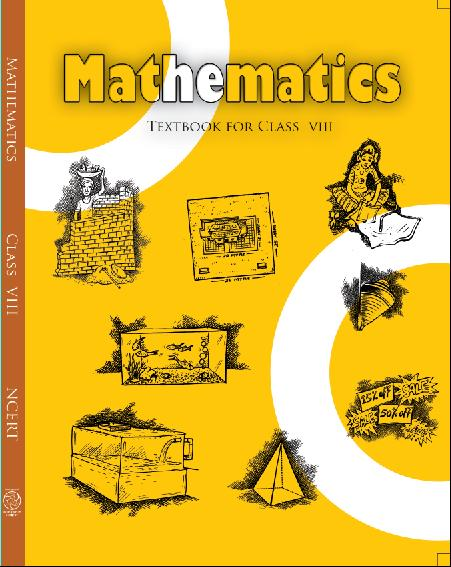## NCERT Solutions for Class 8 Maths Practical Geometry

###### 1. The square READ with RE = 5.1 cm.

Ans. Given:  RE = 5.1 cm.

Steps of construction: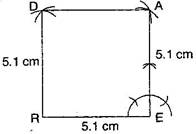(i) Draw RE = 5.1 cm.

(ii) At point E, construct an angle of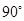and draw an arc of radius 5.1 cm, which intersects at point A.

(iii) At point R, draw an arc of radius 5.1 cm at point A, draw another arc of radius 5.1 cm which intersects the first arc at point D.

It is the required square READ.

NCERT Solutions for Class 8 Maths Exercise 4.5

###### 2. A rhombus whose diagonals are 5.2 cm and 6.4 cm.

Ans. Given:  Diagonals of a rhombus

AC = 5.2 cm and BD = 6.4 cm.

To construct: A rhombus ABCD.

Steps of construction: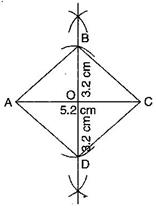(a) Draw AC = 5.2 cm and draw perpendicular bisectors on AC.

(b) Since, diagonals bisect at mid-point O, therefore get half of 6.4 cm, i.e., 3.2 cm.

(c) Draw two arcs on both sides of AC of radius 3.2 cm from intersection point O, which intersects at B and D.

(d) Join AB, BC, CD and DA.

It is required rhombus ABCD.

NCERT Solutions for Class 8 Maths Exercise 4.5

###### 3. A rectangle with adjacent sides of length 5 cm and 4 cm.

Ans. Given:  MN = 5 cm and MP = 4 cm.

To construct: A rectangle MNOP

Steps of construction: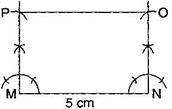(a) Draw a segment MN = 5 cm.

(b) At points M and N, draw perpendiculars of lengths 4 cm and produce them.

(c) Taking centres M and N, draw two arcs of 4 cm each, which intersect P and Q respectively.

(d) Join side PO.

It is required rectangle MNOP.

NCERT Solutions for Class 8 Maths Exercise 4.5

###### 4. A parallelogram OKAY where

OK = 5.5 cm and KA = 4.2 cm.

Ans. Given:  OK = 5.5 cm and KA = 4.2 cm.

To construct: A parallelogram OKAY.

Steps of construction: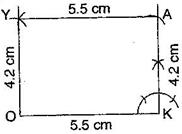(a) Draw a line segment OK = 5.5 cm.

(b) Draw an angle ofat K and draw an arc of radius KA = 4.2 cm, which intersects at point A.

(c) Draw another arc of radius AY = 5.5 cm and at point O, draw another arc of radius 4.2 cm which intersect at Y.

(d) Join AY and OY.

It is the required parallelogram OKAY.

## NCERT Solutions for Class 8 Maths Exercise 4.5

NCERT Solutions Class 8 Mathematics PDF (Download) Free from myCBSEguide app and myCBSEguide website. Ncert solution class 8 Mathematics includes text book solutions from Class 8 Maths Book . NCERT Solutions for CBSE Class 8 Maths have total 16 chapters. 8 Maths NCERT Solutions in PDF for free Download on our website. Ncert class 8 solutions PDF and Maths ncert class 8 PDF solutions with latest modifications and as per the latest CBSE syllabus are only available in myCBSEguide.

## CBSE app for Class 8

To download NCERT Solutions for class 8 Social Science, Computer Science, Home Science,Hindi ,English, Maths Science do check myCBSEguide app or website. myCBSEguide provides sample papers with solution, test papers for chapter-wise practice, NCERT solutions, NCERT Exemplar solutions, quick revision notes for ready reference, CBSE guess papers and CBSE important question papers. Sample Paper all are made available through the best app for CBSE students and myCBSEguide website.Test Generator

Create question paper PDF and online tests with your own name & logo in minutes.myCBSEguide

Question Bank, Mock Tests, Exam Papers, NCERT Solutions, Sample Papers, Notes

### 7 thoughts on “NCERT Solutions for Class 8 Maths Exercise 4.5”

1. It is very useful for me